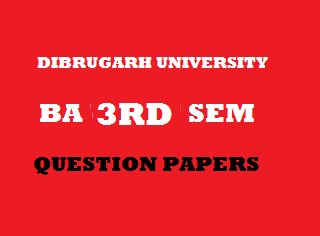## Thursday, January 03, 20192015
(November)
EDUCATION
(General)
Course: 301
(Measurement and Evaluation in Education)
Full Marks: 80
Pass Marks: 32 (Backlog)/24 (2014 onwards)
Time: 3 hours
The figures in the margin indicate full marks for the questions

1. “Evaluation is a tri-polar process.” Who said this?
2. State True or False:
Test is an examination.
1. Write one characteristic of validity.
2. “Intelligence is the ability to carry on abstract thinking.” Who said this?
3. Who was the inventor of Rorschach test?
4. Write the formula of short method of calculating mean.
5. If mean is 30 and median is 30, then what is the value of mode?
6. For computation of coefficient of correlation, who introduced the rank difference method?
2. What is continuous and comprehensive evaluation? Write about the educational significances of measurement and evaluation. 2+2+8=12
3. What is validity of a test? Write briefly about the various types of validity. 2+10=12
4. What is meant by measurement of intelligence? Write briefly the various types of intelligence test.     2+10=12
Or
What is projective method? Discuss about Rorschach ink-bolt test. 2+10=12
5. Write short notes on: 4x4=16
1. Four differences between aptitude and intelligence.
2. Normal probability curve.
3. Sociometric method.
4. Four merits and demerits of mean.
6. Compute mean, median and mode from the following frequency distribution table: 4+4+2=10
 Class interval Frequency 95-9990-9485-8980-8475-7970-7465-6960-6455-5950-5445-4940-44 1245810644231 N = 50

7. Compute coefficient of correlation from the following data using rank difference method and interpret the result:        9+1=10
 Students A B C D E F G H I J Marks in Science 44 36 62 25 10 75 98 78 36 25 Marks in English 53 36 84 68 92 60 36 62 54 37

***